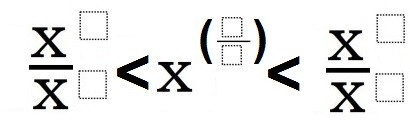# Rational Exponents 4

Directions: Using any number between 1 and 9, fill in the boxes to create a true statement. You may only use a number once.### Hint

What are the properties of exponents? How do they relate?

There are many possible solutions, one is: x^7/x^5 < x^(9/3) < x^8/x^2

Source: Bryan Anderson

## Imaginary Solutions to a Quadratic Equation

Directions: Using the digits 1 to 9 at most one time each, place a digit …

1.2.